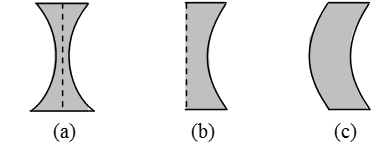Ex﻿

# 4. Light Refraction

#### Spherical Lens

Spherical Lens
Definition : A piece of a transparent medium bounded by at least one spherical surface, is called a spherical lens.
Types : There are two types of spherical lenses:
(i) Convex or Converging Lenses : These are thick in the middle and thin at the edges.Fig. Three types of convex lenses
(a) Double Convex Lens : It has both the surfaces convex.
(b) Plano–Convex Lens : It has one surface plane and the other surface convex.
(c) Concavo–Convex Lens : It has one surface concave and the other surface convex.
(ii) Concave or Diverging Lenses : These are thin in the middle and thick at the edges.
There are three types of concave lenses :Fig. Three types of concave lenses
(a) Double Concave Lens : It has both the surfaces concave. (Fig.)
(b) Plano–Concave Lens : It has one surface plane and the other surface concave. (fig.)
(c) Convexo–Concave Lens : It has one surface convex and the other surface concave. (fig.)

If you want to give information about online courses to other students, then share it with more and more on Facebook, Twitter, Google Plus. The more the shares will be, the more students will benefit. The share buttons are given below for your convenience.
×

#### NTSE Physics (Class X)

• Electricity
• Magnetic Effect of Curent
• Light Reflection
• Light Refraction
• Human Eye & Colourful World
• Source of Energy
• Motion

#### NTSE Physics (Class IX)

• Force & laws of motion
• Work and Energy
• Gravitation
• Sound

SHOW CHAPTERS